# Time clock

What are the angle of the hour hand and the minute hand if it is 0:40?

A =  220 °

### Step-by-step explanation: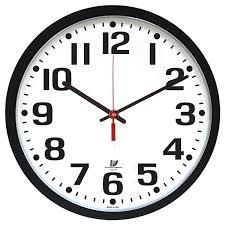Did you find an error or inaccuracy? Feel free to write us. Thank you!Tips to related online calculators
Do you want to convert velocity (speed) units?
Do you want to convert time units like minutes to seconds?

## Related math problems and questions:

• Minute handWhat is the distance the minute hand of the clock travels in 12 minutes, if the diameter of the clock is 30 cm and the hand extends to a distance of 2 cm from the edge of the clock?
• LunchLunch is given to seniors from 12:15 to 12:40 during the Coronavirus pandemic. What angle will the minute hand of clock describe during this time?
• Clock Tower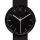What angle is betwenn hands on Clock Tower when show 17 hours and 35 minutes?
• Minute-hand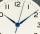How long distance will travel a large (minute) hand on the clock for 48 minutes, if its length is 56 cm?
• Special watchFero bought a special watch on the market. They have only one (minute) hand and a display that shows which angle between the hour and minute hand. How many hours was his watch shown - the minute hand points to number 2; the display shows 125°?
• Speed of clockIn how many minutes will the hour hand pass a straight angle and a right angle?
• HandsThe clock shows 12 hours. After how many minutes will angle between the hour and minute hand 90°? Consider the continuous movement of both hands hours.
• Little hand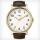What angle shifted little hand on the clock after one hour and 38 minutes?
• Walking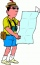Lucie can walk about 3 4/5 miles each hour. How far can she walk in 2 hours 45 minutes?
• Car loop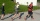The toy car runs at an average speed of 2 m/s. In a minute, it will pass the entire circuit five times. How long is the loop?
• ClocksHow will long path walk the end of the clock's minute-hand long 7 cm for 330 minutes? How long will walk in the same time hour-hand?
• Clock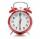What distance will pass end of 8 cm long hour hand for 15 minutes?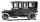Car started at 11:40 at speed 54 km/h from A and to B arrived at 12:20. Determine the distance A and B.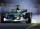Car is traveling 98 km per hour. How long-distance it travel in 1 minute?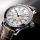Determine size of angle, which takes minute hand for 75 minutes.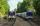Two trains go at each other. First at speed 40 km/h and the second at speed 60 km/h. At what distance will be 30 minutes before they will meet?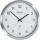In how many minutes describe the minute hand angle 60 degrees?